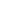Share

# Iteration

Iteration is the repetition of a function or process in a computer program. Iterations of functions are common in computer programming, since they allow multiple blocks of data to be processed in sequence. This is typically done using a "while loop" or "for loop" (see the examples below). These loops will repeat a process until a certain number or case is reached. Recursive functions also use iteration, though instead of repeating a process, the entire function repeats itself.

While loop:   while (x < 30) { ... x++; }
For loop:   for (x=0; x<30; x++) { ... }

A practical example of how iterations are used is a PHP Web page that lists data from a table in a database. In order to display the table on the Web, the function might write each row in HTML using data from the database until the last row of data is reached. In this case, each row of the table would be created by an iteration of the PHP function.

Iterated functions are frequently used in both Web scripts and software programs. They can be simple functions like the one in the example above or complex loops that contain nested loops, which may call additional functions. The amazing thing is that even these complex loops typically only take a fraction of a second to complete.

Published: 2009

Definition from the PC Glossary
https://pc.net/glossary/iteration